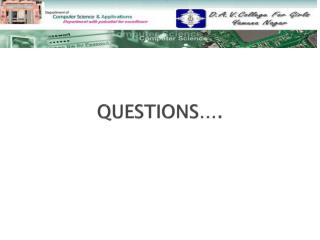DownloadDownload PresentationQUESTIONS….

# QUESTIONS….

Télécharger la présentation## QUESTIONS….

- - - - - - - - - - - - - - - - - - - - - - - - - - - E N D - - - - - - - - - - - - - - - - - - - - - - - - - - -
##### Presentation Transcript

1. QUESTIONS….

2. Differentiate between: • Linear and non linear data structure • Homogenous and non homogenous data structure • Primitive and non primitive data structure • Static and dynamic data structure • What do you mean by sorting? Write the algorithm for selection sort and bubble sort? • Write an algorithm for merging of two arrays? • What is stack? Write the algorithm of push and pop operations?

3. Evaluate the following postfix expression: E=562+*84/- • Write the algorithm of insert and delete operation of the queue? Explain the difference between queue and circular queue? • What is linked list? How can you represent a linked list in memory? • Explain insertion and deletion operations of linked list by giving suitable examples? • Explain the various methods of tree traversal by giving suitable examples?

4. Define: • Graph • Multigraph • Complete graph • Connected graph • Weighted graph • Diagraph